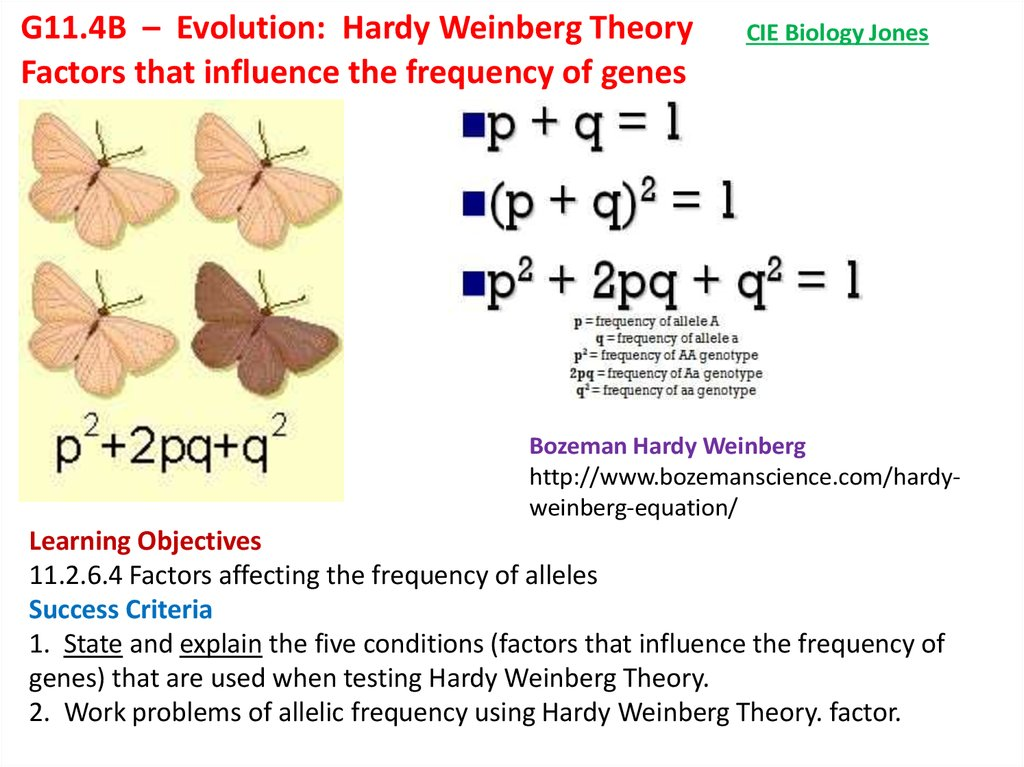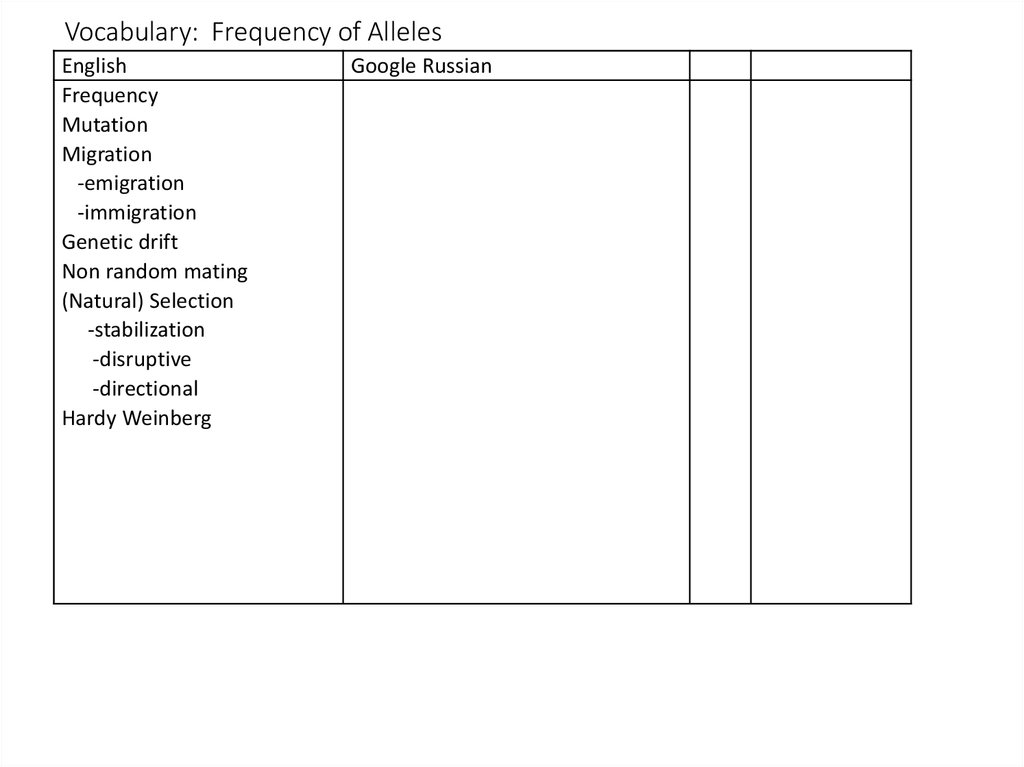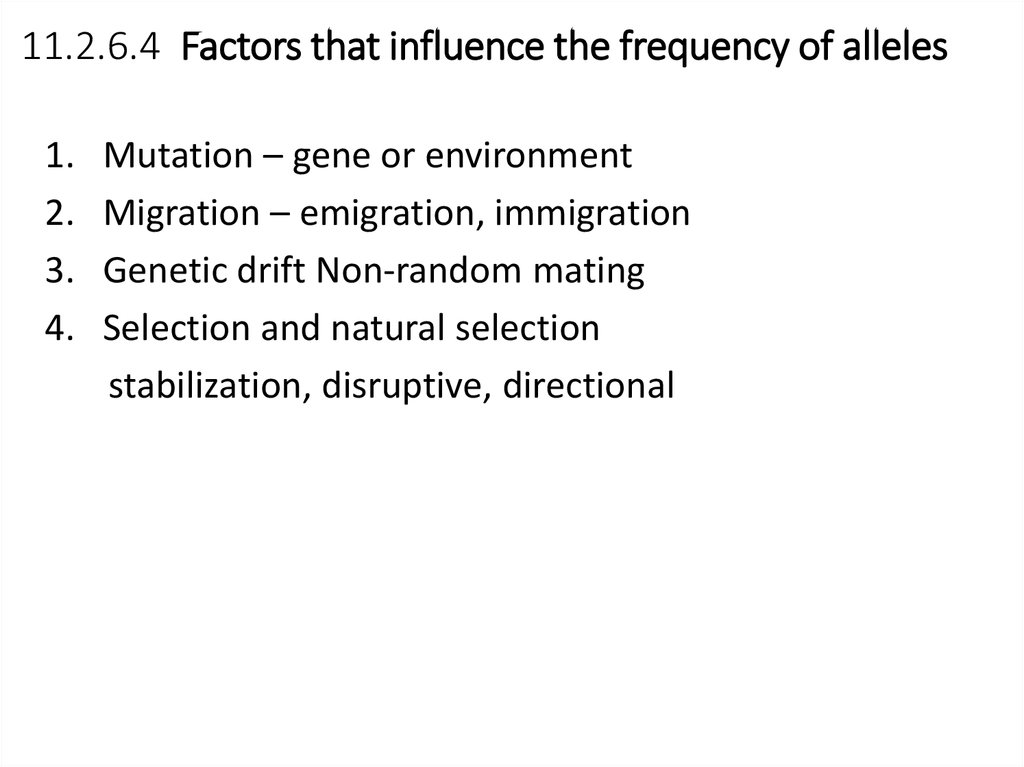# Evolution: Hardy Weinberg Theory Factors that influence the frequency of genes

## 1.

G11.4B – Evolution: Hardy Weinberg Theory
Factors that influence the frequency of genes
CIE Biology Jones
Bozeman Hardy Weinberg
http://www.bozemanscience.com/hardyweinberg-equation/
Learning Objectives
11.2.6.4 Factors affecting the frequency of alleles
Success Criteria
1. State and explain the five conditions (factors that influence the frequency of
genes) that are used when testing Hardy Weinberg Theory.
2. Work problems of allelic frequency using Hardy Weinberg Theory. factor.

## 2. Vocabulary: Frequency of Alleles

English
Frequency
Mutation
Migration
-emigration
-immigration
Genetic drift
Non random mating
(Natural) Selection
-stabilization
-disruptive
-directional
Hardy Weinberg

## 3. 11.2.6.4 Factors that influence the frequency of alleles

1.
2.
3.
4.
Mutation – gene or environment
Migration – emigration, immigration
Genetic drift Non-random mating
Selection and natural selection
stabilization, disruptive, directional

Gene Pool
total number of
genes in a
population at
any one time
4

## 5.

Factors that influence the frequency of alleles
Gene Mutation
A change in the DNA. This can cause
VARIATIONS in individuals which can be
passed to their offspring. Over time
mutations can change a population

## 6.

Factors that influence the frequency of alleles
A light colored allele immigrates
into a population of green colored
alleles
Gene Flow
the physical flow (movement) of alleles into or out of a population
immigration-flow of alleles into a population (added)
emigration- flow of alleles out of a population (lost)
6

## 7. Genetic Drift

Factors that influence the frequency of alleles
Genetic Drift
a species gene pool (number of alleles) will drift
toward genes of traits that allow for more successful
traits.
7

## 8. Non-Random Mating

Factors that influence the frequency of alleles
Non-Random Mating
-Only certain individual organisms
can mate
-
gene pool is small due to
Random
these organisms producing
Mating
more offspring with only their
genes
Non- Random
Mating
evolutionary effects
-less alleles in a population gene
pool
-less chance for evolutionary
change
-more chance of extinction
8

## 9. Directional

Factors that influence the frequency of alleles
Selection
Directional
Disruptive
Stabilizing
Selected for?
Selected against?
Phenotype is selected against /selected for – changing the population
of alleles (genotype)
9

## 10.

Hardy-Weinberg Principle
Starter: The Hardy-Weinberg principle is based on 5 assumptions, use
the image above to guess what these could be. Stretch: Is it truly
representative? Why?

## 11. The principle predicts allele frequencies wont change between generations

• However, this is only true under
certain conditions...
The equations are
used to estimate
allele and genotype
frequencies within
populations
If the frequency of alleles or
genotypes do change then one of
these must have actually occurred

## 12. Hardy-Weinberg

States that the allele frequency for
dominant and recessive alleles remains
the same in a population for many
generations
if the following conditions exist:
1. No mutations can occur.
pp pq
pq qq
2. The population must be large.
3. All mating must be random
4. No migration can occur.
5. All genotypes must be equal
12

## 13. Allele Frequency

how often an form
of a gene shows
up in a population
over several
generations
the number of copies
of a particular allele
divided by the
number of copies of
all alleles at the
genetic place in a
population.
13

## 14. 1. Predicting allele frequency

p+q=1
• You can calculate the frequency of one allele if you know the
frequency of the other allele
• Dominant = p and recessive = q
• For example, red (R) and white (w) flowers
If the frequency of R
is 0.4, then the
frequency of w is?
0.6

## 15. 2. Predicting Genotype frequency

2
p
+ 2pq
2
+q
=1
• You can calculate the frequency of one genotype if you know the
frequency of the others
If the homozygous red phenotype is 0.34, the
• P2 = homozygous dominant
heterozygous phenotype is 0.27, what is the
frequency of the homozygous white flowers?
• 2pq = heterozygous
• q2 = homozygous recessive
• For example, red (R) and white (w) flowers
1 – 0.34 – 0.27 = 0.39

## 16. 3. Using both equations

0.98
0.02
p+q=1
0.957
2
p
0.039
+ 2pq
0.004
2
+q
=1
• Cystic fibrosis (ff genotype) in the UK occurs approximately 1 in
every 2500 births. Calculate the percentage of carriers in the UK.
1. Find q. The homozygous recessive (ff) is q2 in genotype equation.
• 1 / 2500 = 0.004 = q2
• √ of 0.004 = 0.02 = q
2. Find p.
• p + q = 1, so 1 – 0.02 = 0.98 = p
3. Calculate 2pq
• 2pq = 2 x (0.98 x 0.02) = 0.039
• Remember, 2pq is the heterozygous
phenotype (carrier)
• This can be expressed as a percentage
by multiplying by 100 = 3.9%
• 0.039 / 1 X 100 = 3.9 %
• We can also calculate p2 (homo
dominant).

## 17. Question 1

• If 98 out of 200 individuals in a population express
the recessive phenotype, what percent of the
population would you predict would be
heterozygotes?

• 98/200 = (q2)
• 0.49 = q2
• 0.7 = q
•p+q=1
• p = 1 – 0.7
• p = 0.3
• 2pq = 2(0.3)(0.7) = 0.42 = 42% heterozygotes

## 19. Question 2

• 2. Your original population of 200 was hit by a
tidal wave and 100 organisms were wiped out,
leaving 36 homozygous recessive out of the 100
survivors. If we assume that all individuals were
equally likely to be wiped out, how did the tidal
wave affect the predicted frequencies of the
alleles in the population?

• 36/100 = q2
• 0.6 = q
•p+q=1
• p = 0.4
• Heterozygous = 2 (0.4)(0.6) = 0.48 = 48%
• Homozygous dominant = (0.4)(0.4) = 0.16 = 16%

## 21. Question 3

• Lets say that brown fur coloring is dominant to
grey fur coloring in mice. If you have 168 brown
mice in a population of 200 mice........
What is the predicted frequency of
• Homozygous dominants
• Heterozygotes
• Homozygous recessives

200 mice in total
168 = brown = p2 + 2pq
32/200 = grey fur = q2
0.16 = q2
0.4 = q
p = 0.6 (p + q = 1)
• p2 = 0.36 = 36%
• 2pq = 0.48 = 48%
• q2 = 0.16 = 16%

## 23. Question 4

• If 81% of a population is homozygous recessive for
a given trait. Calculate
• Frequency of homozygous dominant
• Frequency of heterozygotes
• Frequency of dominant and recssive alleles

• q2 = 0.81
• q = 0.9
• p = 0.1
• p2 = 0.01
• 2pq = 0.18

## 25. Question 5

• If 51% of the population carries at least one copy
of the recessive allele
• what is the predicted frequency of the population
expressing the dominant phenotype

• 51% = 2pq + q2
• 49% = 0.49 = p2
• 0.7 = p
• 0.3 = q
• p2 + 2pq =
• 0.49 + 0.42 = 0.91 have dominant phenotype

## 27. Question 6

• Albinism is a rare genetically inherited trait that is
only expressed in the phenotype of homozygous
recessive individuals (aa). The most characteristic
symptom is a marked deficiency in the skin and hair
pigment melanin. This condition can occur among
any human group as well as among other animal
species. The average human frequency of albinism in
North America is only about 1 in 20,000.
• calculate the frequency of the dominant allele in
North America
• the frequency of people expressing the normal
phenotype in

• q2 = 1/20,000
• q = 0.0071
• p = 0.9929
• dominant phenotype =
• p2 + 2pq = 0.9859 + 0.1409

## 29. Question 8

• 1 in 1700 US Caucasian new borns have cystic
fibrosis.
• calculate the frequency of the recessive cystic
fibrosis allele and the dominant allele in the
population
• calculate the frequency of non cystic fibrosis
sufferers in the population

• q2 = 1/1700
• q = 0.0243
• p = 0.09757
• p2 + 2pq
• (0.09757)(0.09757) + 2(0.09757)(0.0243)
• 0.9567

## 31. Question 9

• If 9% of an African population is born with a
severe form of sickle-cell anemia (ss), what
percentage of the population will be more
resistant to malaria because they are
heterozygous(Ss) for the sickle-cell gene?

• q2 = 9%
• q2 = 0.09
• q = 0.3
• p = 0.7
• 2pq = 2(0.3)(0.7) = 0.42 = 42%

## 33. Question 10

• The allele y occurs with a frequency of 0.8 in a
population of clams. Give the frequency of
• genotypes YY, Yy, and yy. Show your work!

• The allele y (recessive) has a frequency q = 0.8.
• p + q = 1, then p = 1 – 0.8 = 0.2
• genotype:
• YY genotype frequency = p2 = 0.04
• Yy genotype frequency = 2pq = 0.32
• yy genotype frequency = q2 = 0.64.

## 41. 11.2.6.4 Factors that influence the frequency of alleles

1.
2.
3.
4.
Mutation – gene or environment
Migration – emigration, immigration
Genetic drift Non-random mating
Selection and natural selection
stabilization, disruptive, directional

Gene Pool
total number of
genes in a
population at
any one time
42

## 43.

Factors that influence the frequency of alleles
Gene Mutation
A change in the DNA. This can cause
VARIATIONS in individuals which can be
passed to their offspring. Over time
mutations can change a population

## 44.

Factors that influence the frequency of alleles
A light colored allele immigrates
into a population of green colored
alleles
Gene Flow
the physical flow (movement) of alleles into or out of a population
immigration-flow of alleles into a population (added)
emigration- flow of alleles out of a population (lost)
44

## 45. Genetic Drift

Factors that influence the frequency of alleles
Genetic Drift
a species gene pool (number of alleles) will drift
toward genes of traits that allow for more successful
traits.
45

## 46. Non-Random Mating

Factors that influence the frequency of alleles
Non-Random Mating
-Only certain individual organisms
can mate
-
gene pool is small due to
Random
these organisms producing
Mating
more offspring with only their
genes
Non- Random
Mating
evolutionary effects
-less alleles in a population gene
pool
-less chance for evolutionary
change
-more chance of extinction
46